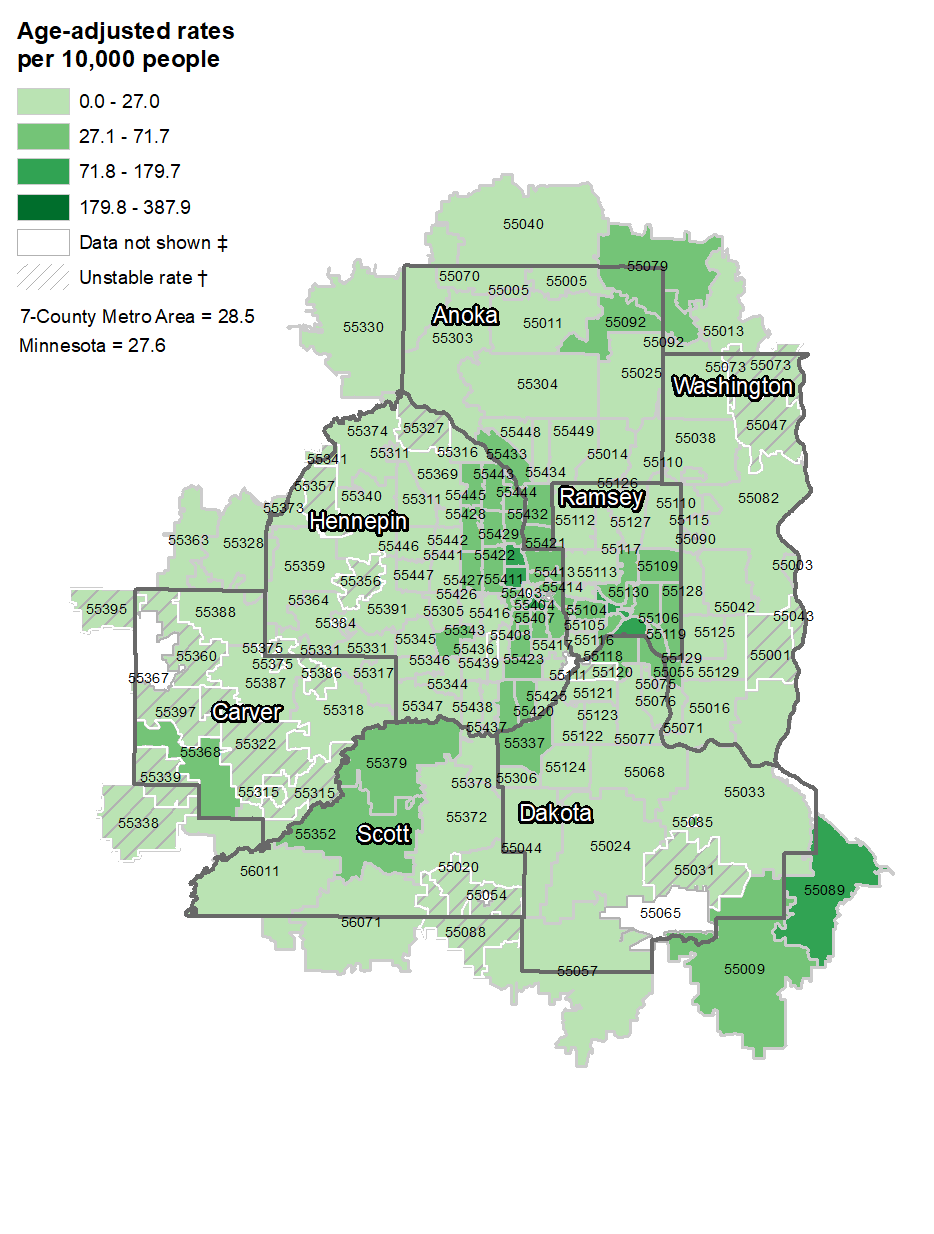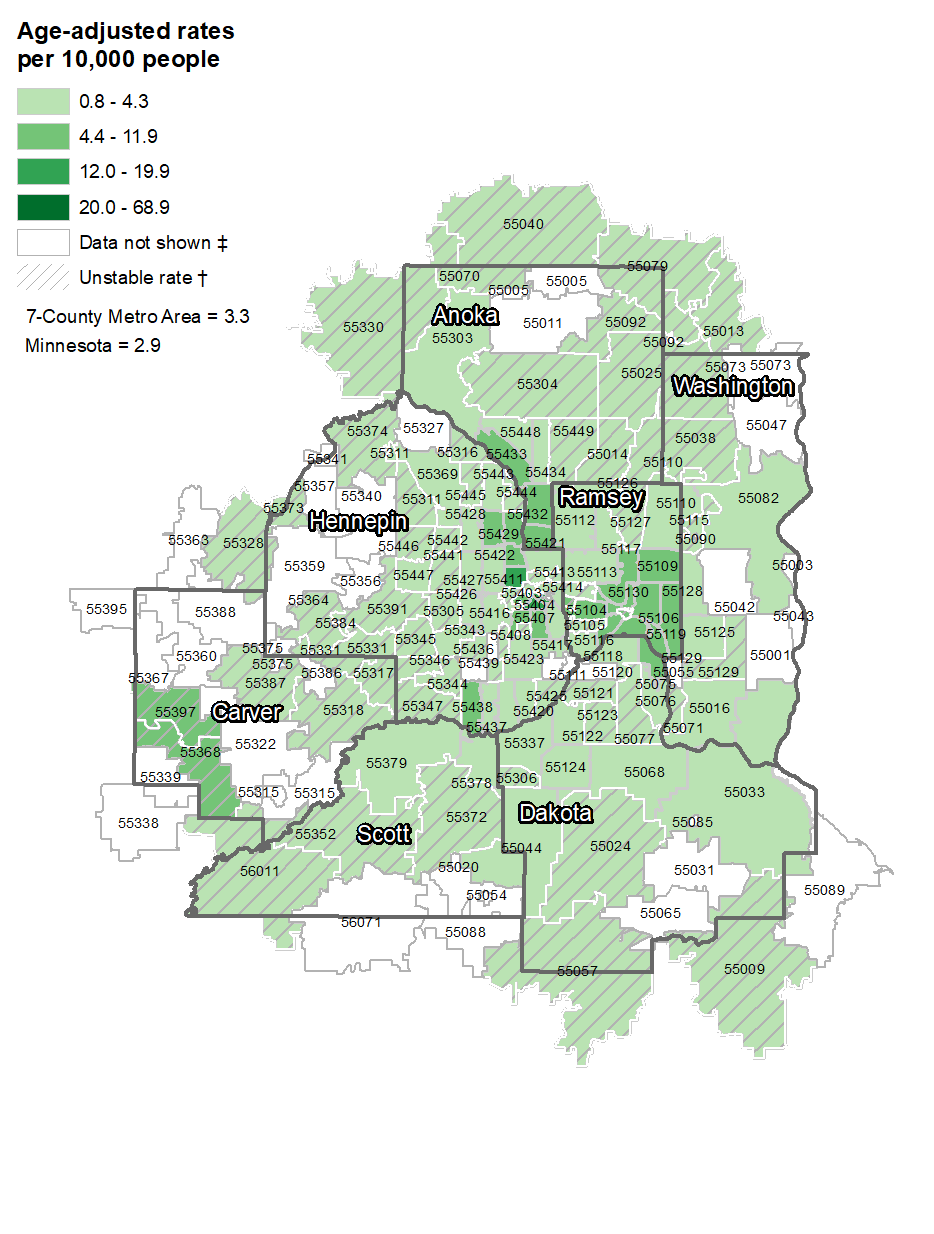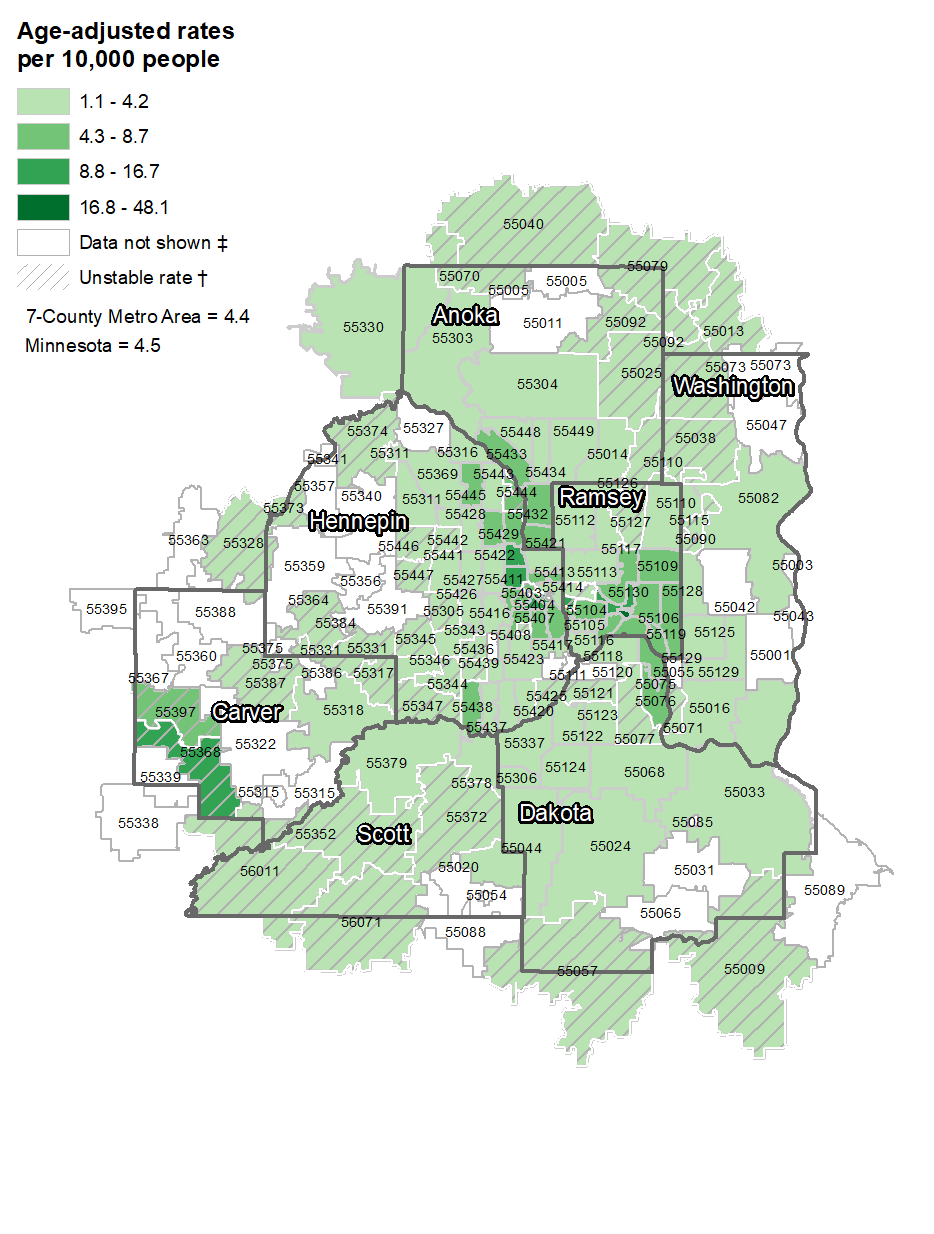Asthma: ZIP Code maps

Asthma ED visits and Hospitalizations by ZIP code in the 7-County Metro Area. These maps supplement the Asthma Hospitalizations Interactive Map.

Asthma emergency department visits† Unstable Rate: rates based on counts from 6 to 20 or with a relative standard error greater than or equal to 30% should be interpreted with caution: the rate may be unstable because it can change dramatically with the addition or subtraction of one case.
‡ Data Not Shown: rates are not calculated when the number of counts is less than or equal to 5, or when the population estimates for that area were not available.† Unstable Rate: rates based on counts from 6 to 20 or with a relative standard error greater than or equal to 30% should be interpreted with caution: the rate may be unstable because it can change dramatically with the addition or subtraction of one case.
‡ Data Not Shown: rates are not calculated when the number of counts is less than or equal to 5, or when the population estimates for that area were not available.† Unstable Rate: rates based on counts from 6 to 20 or with a relative standard error greater than or equal to 30% should be interpreted with caution: the rate may be unstable because it can change dramatically with the addition or subtraction of one case.
‡ Data Not Shown: rates are not calculated when the number of counts is less than or equal to 5, or when the population estimates for that area were not available.

* Unstable Rate: rates based on counts from 6 to 20 or with a relative standard error greater than or equal to 30% should be interpreted with caution: the rate may be unstable because it can change dramatically with the addition or subtraction of one case.
** Data Not Shown: rates are not calculated when the number of counts is less than or equal to 5, or when the population estimates for that area were not available.

Asthma hospitalizations† Unstable Rate: rates based on counts from 6 to 20 or with a relative standard error greater than or equal to 30% should be interpreted with caution: the rate may be unstable because it can change dramatically with the addition or subtraction of one case.
‡ Data Not Shown: rates are not calculated when the number of counts is less than or equal to 5, or when the population estimates for that area were not available.